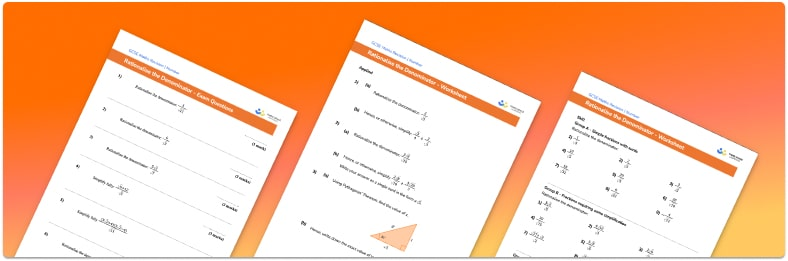# Rationalise The Denominator Worksheet• Section 1 of the rationalise the denominator worksheet contains 27 skills-based rationalise the denominator questions, in 3 groups to support differentiation
• Section 2 contains 3 applied rationalise the denominator questions with a mix of worded problems and deeper problem solving questions
• Section 3 contains 8 foundation and higher level GCSE exam questions
• Answers and a mark scheme for all rationalise the denominator questions are provided
• Questions follow variation theory with plenty of opportunities for students to work independently at their own level
• All questions created by fully qualified expert secondary maths teachers
• Suitable for GCSE maths revision for AQA, OCR and Edexcel exam boards

• This field is for validation purposes and should be left unchanged.

You can unsubscribe at any time (each email we send will contain an easy way to unsubscribe). To find out more about how we use your data, see our privacy policy.

### Rationalise the denominator at a glance

Surds are the square roots of numbers that cannot be simplified. They are used to write irrational numbers precisely for the sake of accuracy in calculations. When surds are written as decimals, they go on forever without any pattern. Irrational numbers cannot be written as fractions with integer numerators and denominators.

Sometimes we might be asked to rationalise the denominator of a fraction. This means finding an equivalent fraction which does not have a surd in the denominator. To rationalise the denominator of a fraction which has one term involving a surd as the denominator, we multiply the numerator and denominator of the fraction by the surd.

To rationalise the denominator when the denominator is made up of a surd and a rational number, we multiply both the numerator and the denominator of the fraction by the conjugate of the denominator. The conjugate is found by changing the sign between the two terms.

Looking forward, students can then progress to additional number worksheets, for example aor aFor more teaching and learning support on Number our GCSE maths lessons provide step by step support for all GCSE maths concepts.

## Do you have KS4 students who need more focused attention to succeed at GCSE?There will be students in your class who require individual attention to help them succeed in their maths GCSEs. In a class of 30, it’s not always easy to provide.

Help your students feel confident with exam-style questions and the strategies they’ll need to answer them correctly with our dedicated GCSE maths revision programme.

Lessons are selected to provide support where each student needs it most, and specially-trained GCSE maths tutors adapt the pitch and pace of each lesson. This ensures a personalised revision programme that raises grades and boosts confidence.

Find out more